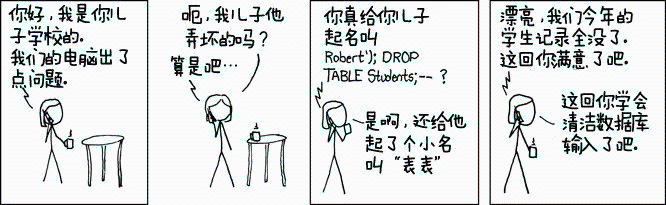# 举世闻名的 SQL 注入是什么？这个漫画告诉你！

6月前 ⋅ 199 阅读

#### 小Hub领读：

SQL注入，XSS，CSRF，都是很常见的攻击手段，也是网站必须要预防的常见攻击，现在已经有很多成熟的预防手段了，不妨大家多多学习学习哈~

• jizhi.im/blog/post/sql_injection_intro### 何谓 SQL 注入

SQL 注入是一种非常常见的数据库攻击手段，SQL 注入漏洞也是网络世界中最普遍的漏洞之一。大家也许都听过某某学长通过攻击学校数据库修改自己成绩的事情，这些学长们一般用的就是 SQL 注入方法。

SQL 注入其实就是恶意用户通过在表单中填写包含 SQL 关键字的数据来使数据库执行非常规代码的过程。简单来说，就是数据「越俎代庖」做了代码才能干的事情。

### SQL 数据库操作示例：

``````import sqlite3
conn = sqlite3.connect('test.db')
conn.executescript('''DROP TABLE IF EXISTS students;
CREATE TABLE students
(id INTEGER PRIMARY KEY AUTOINCREMENT,
name TEXT NOT NULL);''')
students = ['Paul','Tom','Tracy','Lily']

for name in students:
query = "INSERT INTO students (name) VALUES ('%s')" % (name)
conn.executescript(query);
cursor = conn.execute("SELECT id, name from students")
print('IDName')
for row in cursor:
print('{0}{1}'.format(row, row))

conn.close()

``````

### SQL 数据库注入示例：

``````conn = sqlite3.connect('test.db')
name = "Robert');DROP TABLE students;--"
query = "INSERT INTO students (name) VALUES ('%s')" % (name)

conn.executescript(query)
cursor = conn.execute("SELECT id, name from students")
print('IDName')
for row in cursor:
print('{0}{1}'.format(row, row))

conn.close()

``````

``````INSERT INTO students (name) VALUES ('Robert')
INSERT INTO students (name) VALUES ('Robert');DROP TABLE students;

``````

### 如何防止 SQL 注入问题呢？

``````conn = sqlite3.connect('test.db')
name = "Robert');DROP TABLE students;--"
query = "INSERT INTO students (name) VALUES (?)"

conn.execute(query, [name])
cursor = conn.execute("SELECT id, name from students")
print('IDName')
for row in cursor:
print('{0}{1}'.format(row, row))

conn.close()

``````

我有话说: